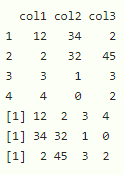# Harmonic Mean in R

• Last Updated : 02 Jun, 2022

In this article, we will see how to find harmonic mean in R programming language.

The harmonic mean is used when an average of rates is required, below is the formula.

Harmonic mean of n numbers x1, x2, x3, . . ., xn can written as below.

Harmonic mean = n / ((1/x1) + (1/x2) + (1/x3) + . . . + (1/xn))

## harmonic.mean() in R

In R, we can compute the harmonic mean by using harmonic.mean() function.

Syntax: harmonic.mean(data)
where, data is an input vector or dataframe.

But this method is available in psych package. so we have to install and import it.

```Install - install.packages("psych")
Import - library("psych")   ```

Example 1:

In this example, we are going to find harmonic mean of vector with 10  elements.

## R

 `# load the library ` `library``(``"psych"``)   ` ` `  `# create vector ` `vector1=``c``(12:21) ` ` `  `# harmonic mean ` `print``(``harmonic.mean``(vector1))`

Output:

` 15.98769`

Example 2:

In this example, we are going to find harmonic mean of  columns in a dataframe. Here we are creating a dataframe with 4 rows and 3 columns and find harmonic mean of three columns. Syntax to find harmonic mean of particular columns in a dataframe.

dataframe\$column

## R

 `# load the library ` `library``(``"psych"``)   ` ` `  `# create dataframe ` `data=``data.frame``(col1=``c``(12,2,3,4), ` `                ``col2=``c``(34,32,1,0), ` `                ``col3=``c``(2,45,3,2)) ` ` `  `# display ` `print``(data) ` ` `  `# harmonic mean of column1  ` `print``(data\$col1) ` ` `  `# harmonic mean of column2 ` `print``(data\$col2) ` ` `  `# harmonic mean of column3 ` `print``(data\$col3)`

Output:My Personal Notes arrow_drop_up
Recommended Articles
Page :